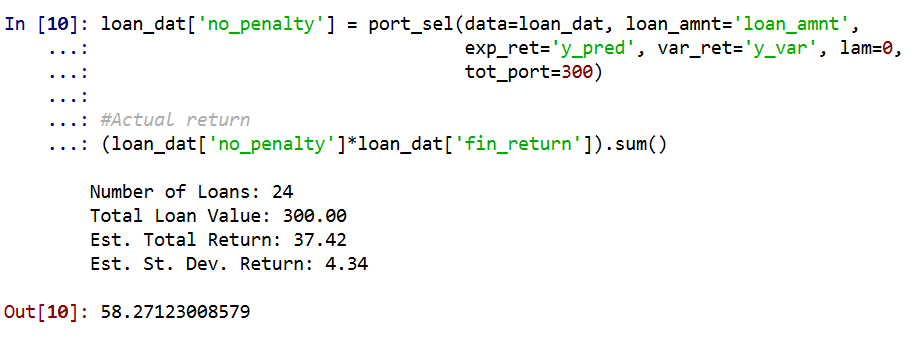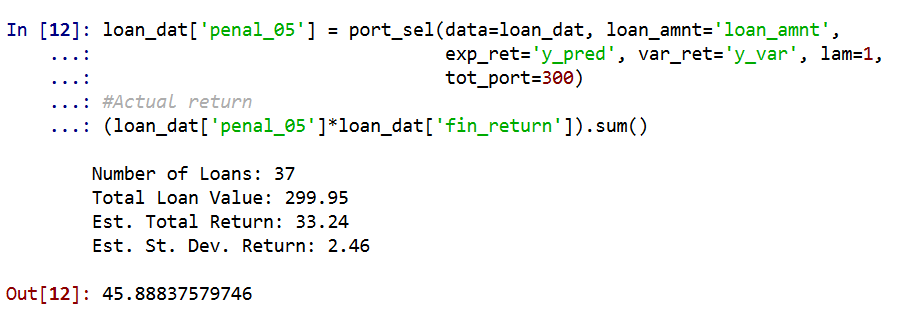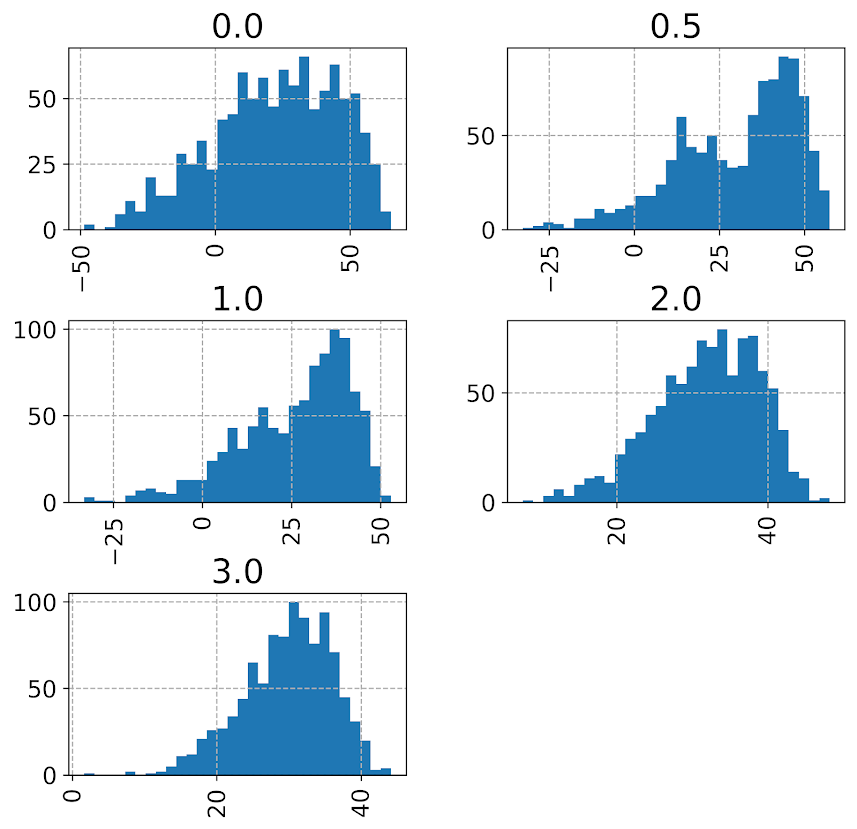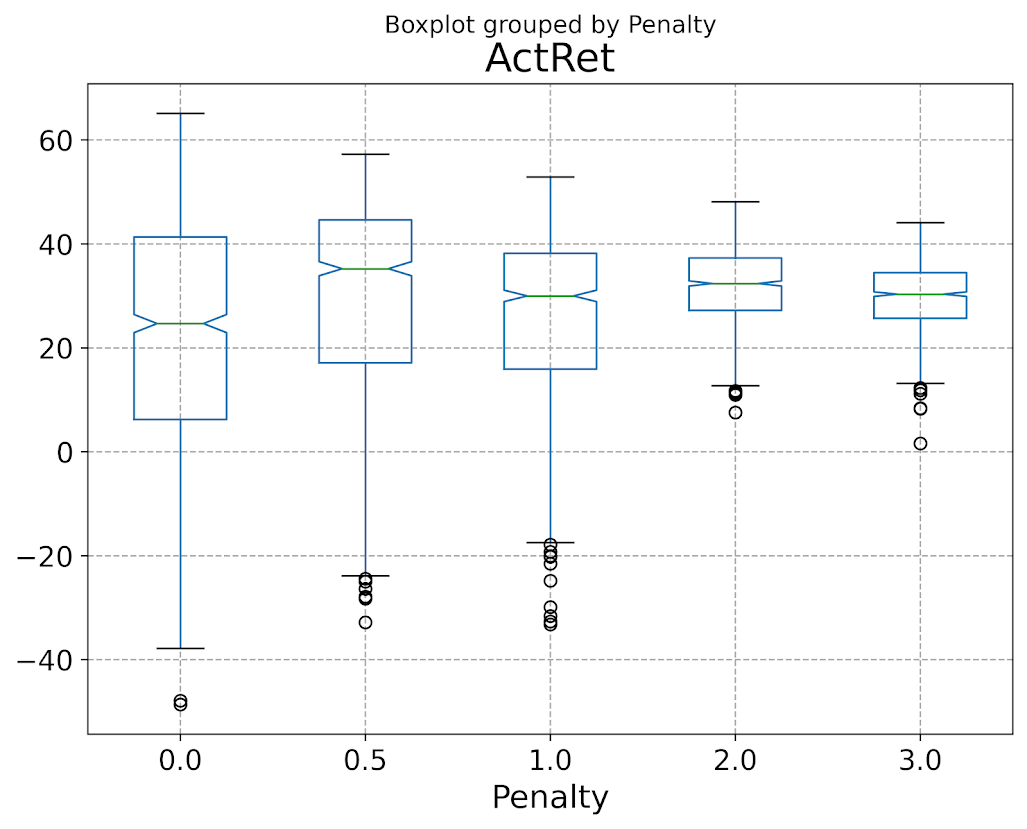# An example of soft constraints in linear programming

Most of the prior examples of linear programming on my site use hard constraints. These are examples where I say to the model, “only give me results that strictly meet these criteria”, like “only select 40 cases to audit”, or “keep the finding rate over 50%”, etc.

There are alternative ways though to tell the model, “I want to select a finding rate over 50%, but still potentially consider those with lower finding rates”. One way to do that is via soft constraints, modifying the objective function directly to penalize (or favor) particular outcomes. For example, say you knew you could translate a 1% finding rate difference over 50% to a value of \$1000. So if our original model is:

``````Maximize Sum{D_i*Return_i}

Subject To
D_i element of (0,1) #decision variables are 0/1
Sum{D_i} = 100       #so select 100 cases``````

We would then place an additional penalty term that looks like this:

``````Maximize Sum{D_i*Return_i} + Sum{D_i*[(prob_i - 0.5)*1000]}

Subject To
D_i element of (0,1)
Sum{D_i} = 100``````

So instead of a subject to constraint that says we need to be over 50% finding rate, we added a second penalty term for solutions that have an under 50% finding rate. So here if the finding rate in the end is 49%, it takes a hit of \$1000 in the objective function. This example is also similar to a bi-objective function, here I just set an exact translation between finding rates and returns, but in practice often you don’t have that exact translation.

It just depends on your situation whether hard constraints or soft constraints make sense. Many situations you can swap one for the other, so different means same ends. For a good example of this, my allocating police resources paper on reducing disproportionate minority contact uses hard constraints (Wheeler, 2020), and George Mohler and colleagues have a very similar paper which uses soft constraints (Mohler et al., 2018). I imagine these will end up being very similar ends, although in that circumstance I prefer my hard constraint approach, as George’s you need to fiddle with the magnitude of the penalty term. Also I don’t tend to like changing the loss function for statistical/machine learning models, I just like changing what you do with the info after you have fit your model (Kleinberg et al., 2018).

Here I provide an example of where I think soft constraints make a bit of sense though. Imagine you have continuous predictive outputs, you need to make a binary yes/no decision among those options, but those predictive outputs also have a variance. An example of where this comes up is if you are making loan decisions, you want your portfolio to have a high return, but you also want to lower the variance of those returns as well.

For a simple example, imagine you are the lending institution and you have the choice between two scenarios:

• Scenario A: lend 1 person \$100,000 with an expected return of \$8,000, with a variance of \$4,000
• Scenario B: lend 2 people \$50,000, with an expected return of each for \$4,000 each, with a variance of \$1,000 for each loan

Since you expect to make the same amount of money under each scenario, option B is preferable if the loans are independent of each other (e.g. one going under does not cause the other to go under). In that case, variances are additive, so the total variance of option B is \$2,000, so has much less volatility than does the A scenario.

(Sorry to my criminology friends, this example is generic but I strain to find a criminal justice example to apply it to. It would not be crazy that you have low volatility vs high volatility hot spots. So you may want to choose a consistent hot spot as oppossed to a fleeting one for an intervention. But I don’t think that will happen in practice quite like that. Choosing among expensive high risk/reward vs inexpensive treatment regimes low risk/reward in corrections settings may also make sense, but that is crazy pie in the sky technocratic given the current state of affairs as well.)

# Example with Lending Club Data

So to illustrate an example with actual data, I’ve provide Python code fitting a predictive model to Lending Club data on loans. (I got the original dataset from Kaggle.) I am just going to highlight some key points here in the blog post. You will need to go to the code to see everything.

First, I’ve been introduced to this dataset as predicting a binary default/no-default. I have code doing that in the code snippet as well, and it performed OK. But it was very uncalibrated as to whether my portfolio made money – so even though the default estimates were pretty well spot on, my portfolios did not make much money. This is because people who default pay back some loans, and also quite a few people in the dataset pay back the loans fast, so the lenders don’t make as much as interest as you would expect at the start of the loan.

So I cut out the middle man and just estimated a random forest model predicting the actual money one made on the loan. I only kept cases that are either `'Fully Paid','Charged Off', 'Default'`, so I don’t model loans that are still ongoing. I end up modeling then the value `total_pymnt - loan_amnt`. You can look into the code to see the variables I included in the model, but one of the neat things about regression random forests is that you can not only get the mean prediction, you can also look at the variance over all the trees. See below a function to do that (in the 01 py file):

``````###############################################################
#Fit random forest model
model = RandomForestRegressor(n_estimators=1000,
random_state=10,
min_samples_leaf=100)
model.fit(train[x_vars], train[y_var])

#Check the predicted vs actual on the test set
y_pred = model.predict(test[x_vars]) #predicted mean
test['y_pred'] = y_pred

#I want an estimate of the variance
def tree_var(X, rf_mod):
per_tree_pred = [pd.Series(tree.predict(X), index=X.index) for tree in rf_mod.estimators_]
pd_res = pd.concat(per_tree_pred, axis=1)
pd_var = pd_res.var(axis=1)
return pd_var

test['y_var'] = tree_var(X=test[x_vars], rf_mod=model)
###############################################################``````

And that predicted value and variance are then what I feed into my subsequent linear programming problem (in the 02 py file). The model in some more text is:

``````Maximize Sum{D_i*(prediction - lambda*variance)}

Subject To:
D element of (0,1)                #decision variables
Sum{D_i*loan_amount_i} <= 300000  #only have so much \$ to loan, so no leveraging
``````

Where lambda is the tuner – higher variances will get higher penalties. So going back to our two loan example, if lambda = 1, scenario A it would be `8000 - 1*4000 = 4000`, and scenario B would be `8000 - 1*2000 = 6000`, so that penalty would choose scenario B over A. Whereas without the penalty the two scenarios are exactly the same.

Since the lambda value is arbitrary, I illustrate the approach selecting portfolios of loans that are a total of \$300,000 (I divided the loans by \$1000 to make the numbers a bit easier to view). This is a totally held out sample of around 5k loans. So you can see my first model (with no constraints):And my second model with a higher lambda value of 1 selects more (smaller) loans, and reduces the variance. You can see since we have the actual outcomes, I can show that both portfolios turned a profit, each above what I predicted. But the standard deviation for second portfolio is cut not quite in half.So you can see in that one selection it worked out OK, but this does not verify that my variance estimates are correct (they are no doubt too small, as you can see the actual returns are way higher than I predicted).

To test them out though I do a simulation. I draw 1000 cases out of those 5000, and then again pick \$300k in loans. I do that process 1000 times under a set of different lambda penalty terms, `[0, 0.5, 1.0, 2.0, 3.0]`. For those simulations, here is the overall distribution of the returns under different penalty terms for the variance.Note that those histograms have different X axes. It is easier to see the moments of the spread in a boxplot:So here you can see each scenario has pretty near the same median return (somewhere around \$30k), but the penalties reduce the variance. The higher penalties end up selecting portfolio’s that always at least make money, whereas the lower penalty terms you do end up losing money in some scenarios.

Unfortunately I did not beat the market in my simple weekend experimentation, so don’t sink a bunch of money into Lending club based on this! The average returns starting from \$300k are something like an annualized rate of return of around 3% (over 3 years) for the smaller simulation pick from 1000. These include loans of 60 months as well though. So even though my linear program with the penalty term did a good job of reducing the risk, this isn’t good enough returns for me to put a bunch of my money into Lending Club.

But there is no doubt improvements both to the modeling as well as the portfolio selection. For modeling I would be tempted to try out a discrete time survival model for payments over time, but that would be more work than I could do in a weekend. (Also I only incorporated the easy continuous variables here I could prepare in just a few minutes, so maybe more feature engineering would boost my results.)

I could also adapt the linear program to take into account covariance between the loans, but not sure how to estimate them (a multi-level model perhaps?). You also may want to do some sort of conditional value at risk approach in the linear program, say instead of piping in the variance from random forests, count up how often you lose money and put that as a penalty or constraint on the system.